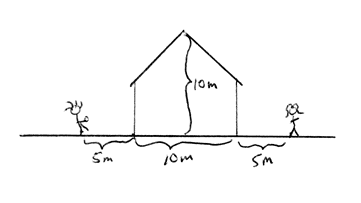# X and y components of initial velocity

Jaco Leo
Warning! Be sure to retain and fill out all sections of the homework template.

## Homework Statement

You’re 5.00 m from the left-hand wall of the house shown in the drawing, and you want to throw a ball to a friend 5.00 m from the right-hand wall. You throw the ball so that at the peak of its trajectory it just clears the top of the roofa. What was the y-component of the initial velocity?

b. What was the x-component of the initial velocity?

Not sure how to go about this problem since it doesn't tell me anything other than the distances, no angles or velocities, any help would be great.

Gold Member
2022 Award
The problem statement tells you everything you need to know to solve it. Do you understand ballistic trajectories under the influence of gravity?

Mentor
Not sure how to go about this problem since it doesn't tell me anything other than the distances, no angles or velocities, any help would be great.
The angle is ##\alpha##, the initial velocity is ##v##. Now you can use both to set up your equations.
You'll be able to calculate numbers for those two quantities later.

Simply introducing variables for unknown quantities is a very useful technique in many problems.

Jaco Leo
still don't really understand how to properly answer the questions. How do i actually figure out the x and y components based on the information? Do I set it up in a Axi + Ayj type of format?

Mentor
With the angle and velocity, you can calculate the horizontal and vertical velocity components, and then you can check the trajectory. What has to be satisfied to give the correct flight path?

Gold Member
2022 Award
Did you read post #2? Do you have an answer to my question?

Jaco Leo
With the angle and velocity, you can calculate the horizontal and vertical velocity components, and then you can check the trajectory. What has to be satisfied to give the correct flight path?

the total distance seems to be 20m and there will always been a downward force of 9.8m/s^2 which is gravity. I just don't know how to start to find the velocity, and the only angle that i see is maybe a 90 degree angle? But I'm not sure about that either.

Mentor
I just don't know how to start to find the velocity
You know the velocity, it is v. How far will the object fly, given the known velocity and angle? You have all the relevant formulas in your textbooks and you should have them in your notes as well.

It does not matter if you can see the full solution path now - just trust me that starting with the hints I gave you is a good way.

Jaco Leo
You know the velocity, it is v. How far will the object fly, given the known velocity and angle? You have all the relevant formulas in your textbooks and you should have them in your notes as well.

It does not matter if you can see the full solution path now - just trust me that starting with the hints I gave you is a good way.

but there is no known velocity or angle in the problem?

Gold Member
2022 Award
but there is no known velocity or angle in the problem?

Yeah, that's why I keep asking you if you understand ballistic trajectories in gravity. I guess you've answered that question. Perhaps you would find it useful to look them up, which is what I kept hinting at.

Mentor
but there is no known velocity or angle in the problem?
Do you understand the concept of variables?

dean barry
You know the vertical height = 10 m and with a value for g, you can figure the vertical component of the launch velocity and the time taken to reach the zenith (top of the roof)
With the time you can figure the (constant) horizontal velocity required.

Jaco Leo
Here's what I came up with so far. I know sin of the angle = Vy/V and cos of the angle = Vx/V. Then do some algebra to get Vy = Vsin of angle and Vx=Vcos of angle. But since It doesn't list a velocity in the problem, how are you actually suspose to get a value for the x and y components of the initial velocity? Or can you with the given data? Would it just have to be a sort of equation like I figured out before? Can somebody please tell me the answer to this and explain it to me, because all I've gotten now are just very vague points about how i should look back at this and that, an answer is the only thing that would help me actually understand this problem. Thank you!

Gold Member
How fast do you need to throw the ball for it to go 10 meters up with an acceleration of g?

Jaco Leo
How fast do you need to throw the ball for it to go 10 meters up with an acceleration of g?
do you use kinematic equations to figure this out then?

Mentor
do you use kinematic equations to figure this out then?
What else?

We keep telling you to get started, and you keep complaining that you don't see how this will finally lead to your solution. It is pointless to continue the thread like that. Feel free to send me a message once you got started (see all previous replies how to do that), then I will re-open this thread.

•phinds